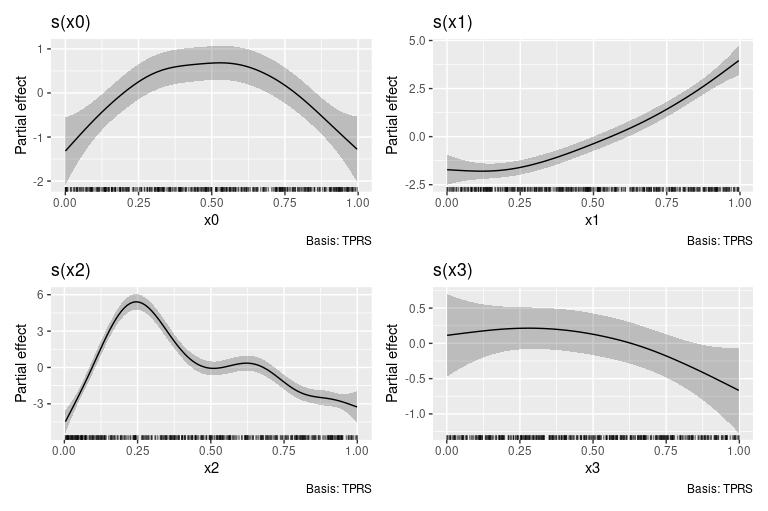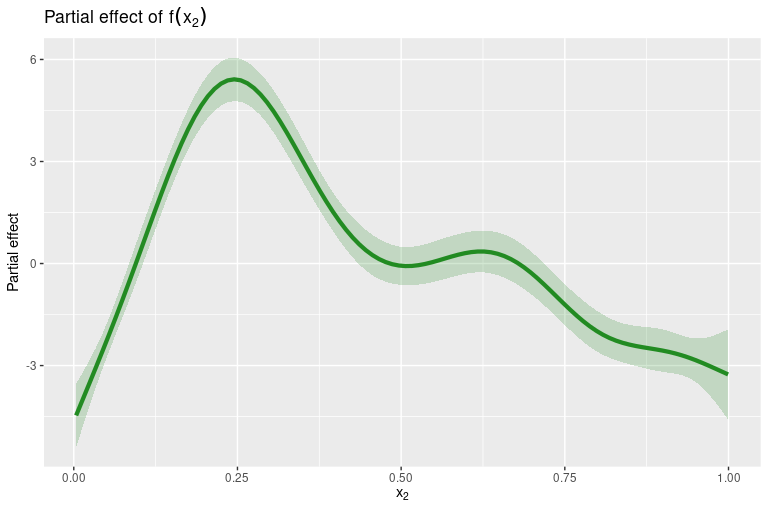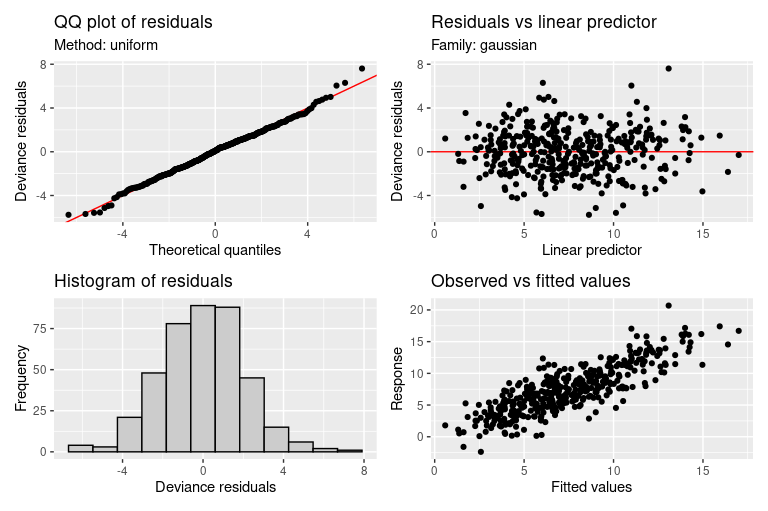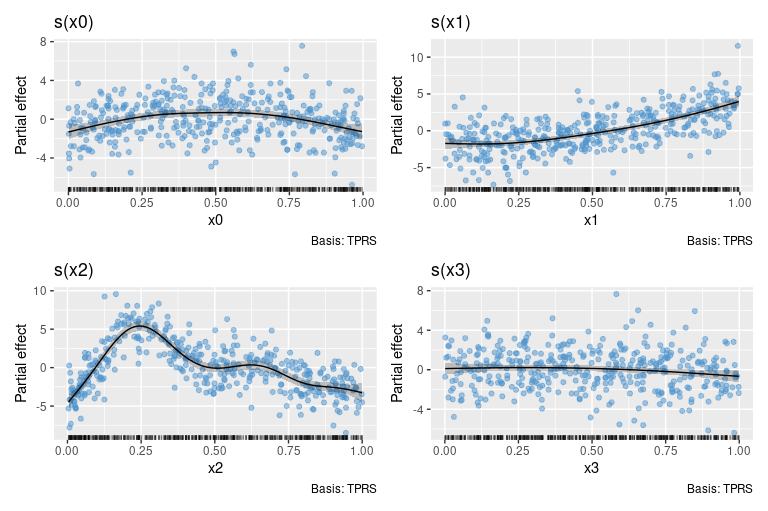# Get started with gratia

library("gratia")
library("mgcv")

gratia is a package to make working with generalized additive models (GAMs) in R easier, including producing plots of estimated smooths using the ggplot2 📦.

This introduction will cover some of the basic functionality of gratia to get you started. We’ll work with some classic simulated data often used to illustrate properties of GAMs

df <- data_sim("eg1", seed = 42)
df
#> # A tibble: 400 × 10
#>        y    x0     x1    x2    x3     f    f0    f1     f2    f3
#>    <dbl> <dbl>  <dbl> <dbl> <dbl> <dbl> <dbl> <dbl>  <dbl> <dbl>
#>  1  2.99 0.915 0.0227 0.909 0.402  1.62 0.529  1.05 0.0397     0
#>  2  4.70 0.937 0.513  0.900 0.432  3.25 0.393  2.79 0.0630     0
#>  3 13.9  0.286 0.631  0.192 0.664 13.5  1.57   3.53 8.41       0
#>  4  5.71 0.830 0.419  0.532 0.182  6.12 1.02   2.31 2.79       0
#>  5  7.63 0.642 0.879  0.522 0.838 10.4  1.80   5.80 2.76       0
#>  6  9.80 0.519 0.108  0.160 0.917 10.4  2.00   1.24 7.18       0
#>  7 10.4  0.737 0.980  0.520 0.798 11.3  1.47   7.10 2.75       0
#>  8 12.8  0.135 0.265  0.225 0.503 11.4  0.821  1.70 8.90       0
#>  9 13.8  0.657 0.0843 0.282 0.254 11.1  1.76   1.18 8.20       0
#> 10  7.51 0.705 0.386  0.504 0.667  6.50 1.60   2.16 2.74       0
#> # … with 390 more rows

and the following GAM

m <- gam(y ~ s(x0) + s(x1) + s(x2) + s(x3), data = df, method = "REML")
summary(m)
#>
#> Family: gaussian
#>
#> Formula:
#> y ~ s(x0) + s(x1) + s(x2) + s(x3)
#>
#> Parametric coefficients:
#>             Estimate Std. Error t value Pr(>|t|)
#> (Intercept)   7.4951     0.1051   71.35   <2e-16 ***
#> ---
#> Signif. codes:  0 '***' 0.001 '**' 0.01 '*' 0.05 '.' 0.1 ' ' 1
#>
#> Approximate significance of smooth terms:
#>         edf Ref.df      F  p-value
#> s(x0) 3.425  4.244  8.828 8.78e-07 ***
#> s(x1) 3.221  4.003 67.501  < 2e-16 ***
#> s(x2) 7.905  8.685 67.766  < 2e-16 ***
#> s(x3) 1.885  2.359  2.642   0.0636 .
#> ---
#> Signif. codes:  0 '***' 0.001 '**' 0.01 '*' 0.05 '.' 0.1 ' ' 1
#>
#> R-sq.(adj) =  0.685   Deviance explained = 69.8%
#> -REML = 886.93  Scale est. = 4.4144    n = 400

## Plotting

gratia provides the draw() function to produce plots using the ggplot2 📦. To draw the estimated smooths from the GAM we fitted above, use

draw(m)This is intended as reasonable overview of the estimated model, but it offers limited option to modify the resulting plot. If you want full control, you can obtain the data used to create the plot above with smooth_estimates()

sm <- smooth_estimates(m)
sm
#> # A tibble: 400 × 9
#>    smooth type  by       est    se       x0    x1    x2    x3
#>    <chr>  <chr> <chr>  <dbl> <dbl>    <dbl> <dbl> <dbl> <dbl>
#>  1 s(x0)  TPRS  <NA>  -1.32  0.390 0.000239    NA    NA    NA
#>  2 s(x0)  TPRS  <NA>  -1.24  0.365 0.0103      NA    NA    NA
#>  3 s(x0)  TPRS  <NA>  -1.17  0.340 0.0204      NA    NA    NA
#>  4 s(x0)  TPRS  <NA>  -1.09  0.318 0.0304      NA    NA    NA
#>  5 s(x0)  TPRS  <NA>  -1.02  0.297 0.0405      NA    NA    NA
#>  6 s(x0)  TPRS  <NA>  -0.947 0.279 0.0506      NA    NA    NA
#>  7 s(x0)  TPRS  <NA>  -0.875 0.263 0.0606      NA    NA    NA
#>  8 s(x0)  TPRS  <NA>  -0.803 0.249 0.0707      NA    NA    NA
#>  9 s(x0)  TPRS  <NA>  -0.732 0.237 0.0807      NA    NA    NA
#> 10 s(x0)  TPRS  <NA>  -0.662 0.228 0.0908      NA    NA    NA
#> # … with 390 more rows

which will evaluate all smooths are unevenly spaced values over the range of the covariate(s). If you want to evaluate only selected smooths, you can specify which via the smooth argument. This takes the smooth labels which are the names of the smooths as they are known to mgcv. To list the labels for the smooths in use

smooths(m)
#>  "s(x0)" "s(x1)" "s(x2)" "s(x3)"

To evaluate only $$f(x_2)$$ use

sm <- smooth_estimates(m, smooth = "s(x2)")
sm
#> # A tibble: 100 × 6
#>    smooth type  by        est    se      x2
#>    <chr>  <chr> <chr>   <dbl> <dbl>   <dbl>
#>  1 s(x2)  TPRS  <NA>  -4.47   0.476 0.00359
#>  2 s(x2)  TPRS  <NA>  -4.00   0.406 0.0136
#>  3 s(x2)  TPRS  <NA>  -3.53   0.345 0.0237
#>  4 s(x2)  TPRS  <NA>  -3.06   0.295 0.0338
#>  5 s(x2)  TPRS  <NA>  -2.58   0.263 0.0438
#>  6 s(x2)  TPRS  <NA>  -2.09   0.250 0.0539
#>  7 s(x2)  TPRS  <NA>  -1.59   0.253 0.0639
#>  8 s(x2)  TPRS  <NA>  -1.08   0.264 0.0740
#>  9 s(x2)  TPRS  <NA>  -0.564  0.278 0.0841
#> 10 s(x2)  TPRS  <NA>  -0.0364 0.289 0.0941
#> # … with 90 more rows

Then you can generate your own plot using the ggplot2 package, for example

library("ggplot2")
library("dplyr")
sm %>%
ggplot(aes(y = est, x = x2)) +
geom_ribbon(aes(ymin = lower_ci, ymax = upper_ci),
alpha = 0.2, fill = "forestgreen") +
geom_line(colour = "forestgreen", size = 1.5) +
labs(y = "Partial effect",
title = expression("Partial effect of" ~ f(x)),
x = expression(x))## Model diagnostics

The appraise() function provides standard diagnotic plots for GAMs

appraise(m)The plots produced are (from left-to-right, top-to-bottom),

• a quantile-quantile (QQ) plot of deviance residuals,
• a scatterplot of deviance residuals against the linear predictor,
• a histogram of deviance residuals, and
• a scatterplot of observed vs fitted values.

Adding partial residuals to the partial effect plots produced by draw() can also help diagnose problems with the model, such as oversmoothing

draw(m, residuals = TRUE)